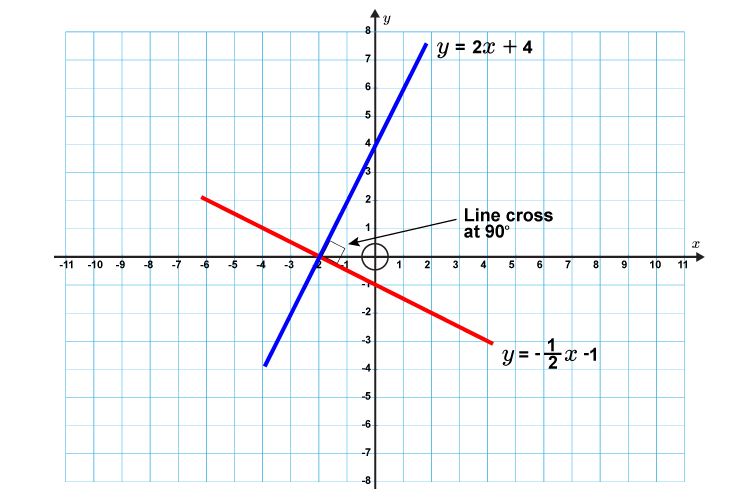# Examples of perpendicular lines

1.  Are these straight lines perpendicular?The formula for each line is:

y = 2x + 4

And         y=-1/2x-1

And  y=mx+c where m is the gradient

The test for perpendicular

m_1\times\m_2=-1

2\times-1//2=?

2\times-1//2=-1

Yes these lines are perpendicular.